# 论文阅读

## DeepRoad, GAN-Based Metamorphic Testing and Input Validation Framework for Autonomous Driving Systems

Posted by MY on September 6, 2018

# 背景

1. DeepTest和DeepXplore生成的图像失真很严重，如下图，有的有黑点，有的加黑线条。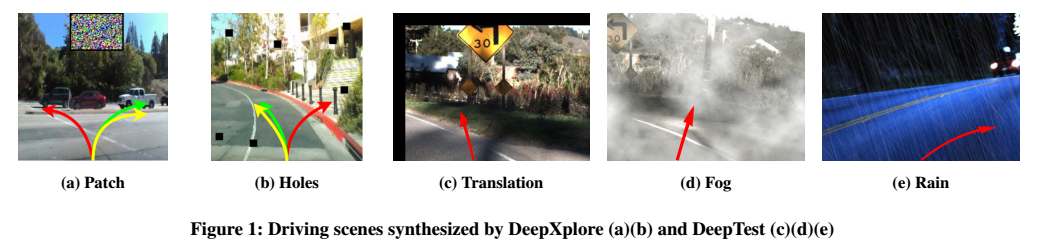而本文生成的就很逼真：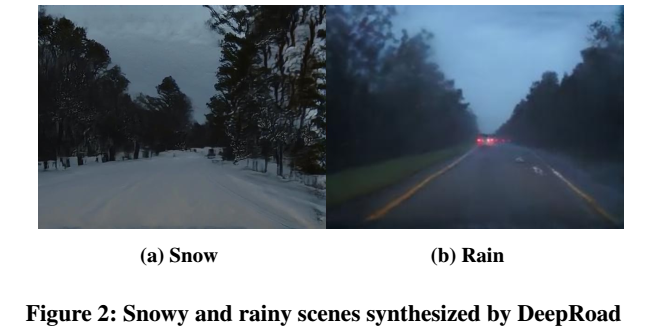2. 当前基于DNN的系统缺乏验证输入，并因此可能会导致系统漏洞。例如，假设基于DNN的自动驾驶系统在仅包括晴天驾驶场景图像的数据集上训练，对于系统未经训练的输入（例如雨天驾驶场景的图像），系统很可能输出错误的控制信号，这会导致危险。
针对以上的问题，本文的贡献点为：

• 本文提出了第一种基于GAN的蜕变测试方法，用于生成具有各种天气条件的驾驶场景，用于检测自动驾驶系统的不一致行为。
• 本文提出了一种新方法来验证基于DNN的自动驾驶系统的输入。我们证明训练和输入图像的高级特征之间的距离可用于验证输入的安全性。

# 基于DNN的自动驾驶系统的蜕变测试

## 1. 蜕变测试

蜕变测试（MT）已被广泛用于自动生成测试以检测软件故障。MT的优势在于它能够通过蜕变关系（MR）自动解决测试先知的问题。测试先知是指，为了测试一个程序，我们需要按照需求给出应当得到的结果。
令$p$为将程序输入映射到程序输出的数学表示，$i$为输入，$o$为输出（$p[i]=o$）。假设$f_{I}$和$f_{O}$分别是用于变换输输入域和输出域的两个特定函数，并且它们满足以下MR公式：&\forall i,p[f_{I}(i)]=f_{O}(p[i])& 　　例如，假设我们有一个计算sin函数的程序(输入值为弧度)，一般情况下我们可以利用一些特殊值去进行测试，比如sin(0)=0，sin(Pi/2)=1 等。但是假设我们有这么一个测试用例sin(2)，其程序结果为0.909，那我们如何能判定这个结果的正确性呢？这种情况下，我们可以根据一些已知的MR去产生后续测试用例进行测试，比如sin(x)=-sin(-x)，sin(x)=sin(pi-x)(这就是MR)等。如果有任何违反以上关系的情况发生，那么我们就可以认定程序中存在错误。
将这种思想应用到自动驾驶测试中如下：将DNN表示为基于DNN的自动驾驶系统，其连续地将每个图像映射到预测的车辆所需要转向的角度的信号（例如，向左转15°）。 在给定原始图像流I的情况下可以定义一个MR，各种图像变换T可以简单地改变道路场景而不影响每个图像的预测（例如，在不同的天气条件下，在同一条道路上，预测的转弯方向应该大致相同）。这样测试DNN的MR可以如下形式化： &\forall i\in I \wedge \forall i\in T,DNN[t(i)]=DNN[i]&

## 2. 基于DNN的道路场景转换

为了通过自动生成各种真实世界的道路场景，在这项工作中，作者利用了UNIT。这是最近发布的基于DNN的方法，基于生成对抗网络（GAN）和变分自动编码器（VAE）执行无监督的图像到图像转换。UNIT的思想是假设两个图像包含相同的内容但位于不同的域中，它们应该在共享的一个潜在空间中具有相同的表示。因此，给定来自一个域（例如，晴天驾驶场景）的新图像，UNIT可以在另一个域（例如，下雨的驾驶场景）中自动生成其对应的版本。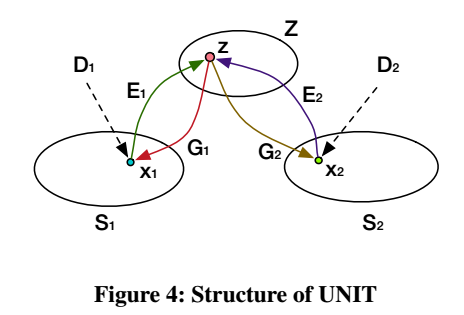其中，$S_{1}$和$S_{2}$表示两个不同的域（例如，晴天和多雨的驾驶场景），$E_{1}$和$E_{2}$表示两个自动编码器，它们将图像从$S_{1}$和$S_{2}$投影到共享的潜在空间$Z$。假设$x_{1}$和$x_{2}$是共享相同内容的成对图像。$E_{1}$和$E_{2}$将它们编码为相同的潜在向量$z$，并且它可以分别由两个域的特定生成器$G_{1}$和$G_{2}$转换回$S_{1}$和$S_{2}$。$D_{1}$和$D_{2}$是两个鉴别器，它们分别检测图像是否属于$S_{1}$和$S_{2}$。具体地说，期望它们区分输入图像是从目标域（例如真实图像）采样还是由生成器（例如合成图像）产生的。基于自动编码器和生成器，UNIT可用于在两个域之间转换图像。例如，图像$x_{1}$可以通过$G_{2}(E_{1}(x_{1}))$变换至$S_{2}$域内。 　　在UNIT中，所有D, E和G都是为神经网络，UNIT的学习优化的目标有如下几个：

• VAE loss：最大限度地减少每个$< E_{i},G_{i}>$的图像重建损失
• GAN loss：在每个$< G_{i},D_{i}>$中达到minimax的均衡点，其中$D_{i}$旨在区分图像以确定它们是从域$S_{i}$采样还是由$G_{i}$生成
• Cycle-consistency loss：最小化每个$< E_{i},G_{j},E_{j},G_{i}>$的循环重建损失，其中$x_{1}$希望等于$G_{1}(E_{2}(G_{2}(E_{1}(x_{1}))))$，$x_{2}$希望等于$G_{2}(E_{1}(G_{1}(E_{2}(x_{2}))))$
• 总的loss如下并使用SGD进行优化: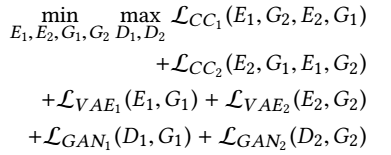## 3. $DeepRoad_{MT}$的框架

$DeepRoad_{MT}$先从两个目标域（例如，分别在晴天和下雪天气下的驾驶场景的数据集）中获取未配对的训练图像，并利用UNIT通过优化上面介绍的损失函数将两个域投影到相同的潜在空间。当训练过程完成后，$DeepRoad_{MT}$使用训练好的模型将晴天驾驶场景的整个数据集转换为下雪天气的驾驶场景。$DeepRoad_{MT}$将每对真实和合成驾驶场景图像馈送到被测自动驾驶系统，即DNN，并比较它们的预测结果$DNN[t(i)]$和$DNN(i)$以检测任何不一致的行为。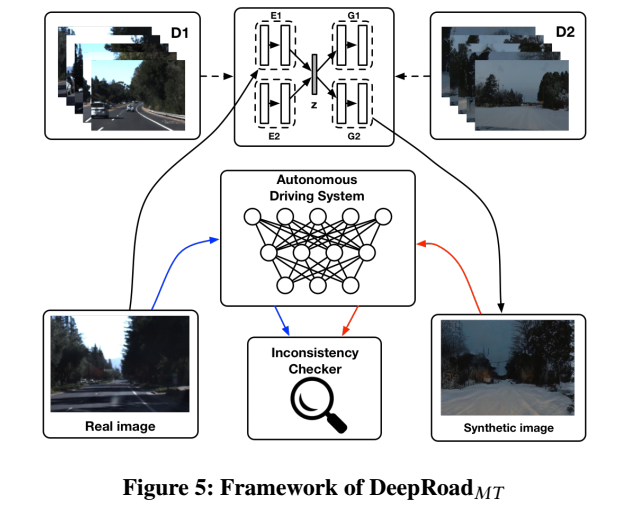# 基于DNN的自主驾驶系统的输入验证

## 1. DNN输入的验证

输入验证（IV）的目标是确保系统只能接受正确的数据，并且在执行之前应该拒绝格式错误的数据。例如，我们可以定义IV标准如下：第一种是输入数据应该是大小为640 * 480的任何RGB图像；第二种是任何输入数据都应该存在于训练数据集中以保证正确性。 但是，由于第一个标准太弱而无法提高系统稳健性，而第二个标准太强，导致系统缺乏通用性，因此都不适合。
本文基于PAC（Probably Approximately Correct）理论来定义基于DNN的程序的IV标准。直观上来说，IV标准应该如下：&Pr_{x\sim D}(x=i)>\theta& $D$是数据集，$x$是从中采样的输入。这意味着从$D$采样输入$i$的概率应该大于预定阈值$\theta$。 否则，系统拒绝在i上预测。 然而，这种定义对于图像数据是不易处理的，因为图像数据是高度维度的并且它们的分布难以明确地表示。为了解决这个问题，本文将图像数据投影到低维空间，并使用输入和训练数据之间的距离来替换$Pr_{x\sim D}(x=i)$。根据Manifold Learning theory，由$D$生成的图像可以嵌入到非线性低维流形$M_{D}$中。假设输入数据i是从D中采样的，其投影$i_{p}$应包含在$M_{D}$中。我们可以计算$i_{p}$和训练数据之间的最小距离，以验证$i_{p}$是否属于$M_{D}$。IV标准重新定义如下：&\underset{j}{min}\left | h(i)-h(j) \right |_{2}<\theta ‘& 其中$\left | \cdot \right |$是$L_{2}$范式，$h(\cdot)$表示所需的非线性投影，$\theta ‘$表示输入验证的预定阈值。如果输入满足该公式，则它将由DNN处理以进行预测，否则将被拒绝预测。

## $DeepRoad_{IV}$的框架

$DeepRoad_{IV}$将投影$h(\cdot)$分为两部分：非线性变换和降维。对于非线性变换，$DeepRoad_{IV}$应用VGGNet，用于从每个图像中提取高级特征。$DeepRoad_{IV}$还生成样式信息。这些样式信息旨在捕获图像的纹理，并通过特征关联来定义。对于降维来说，本文应用主成分分析（PCA）技术来减少输入和训练数据的特征维度。
$DeepRoad_{IV}$先将训练图像和在线驾驶图像作为输入，并使用VGGNet提取其内容和样式特征。如下图所示,$DeepRoad_{IV}$将雪景的图像输入到VGGNet，并选择卷积层conv 4_2和conv 5_3分别提取内容和样式特征。具体来说，彩色网格F 4_2和F 5_3表示从VGGNet中提取的内容特征，样式特征G 5_3由文中的公式７计算。然后，矩阵F 4_2和G 5_3被观察并连接到特征向量v。$DeepRoad_{IV}$使用相同的方法处理所有图像数据，并且将特征向量组成矩阵X。在第二步中，$DeepRoad_{IV}$应用PCA来减少特征维度。在下图中，我们将目标维度设置为2。处理后的数据Y呈现在2D平面上，其中蓝色和红色节点分别表示训练的图像和在线驾驶图像。最后，$DeepRoad_{IV}$计算训练数据和每个在线驾驶图像之间的最小距离，并拒绝预测距离大于某个阈值的图像。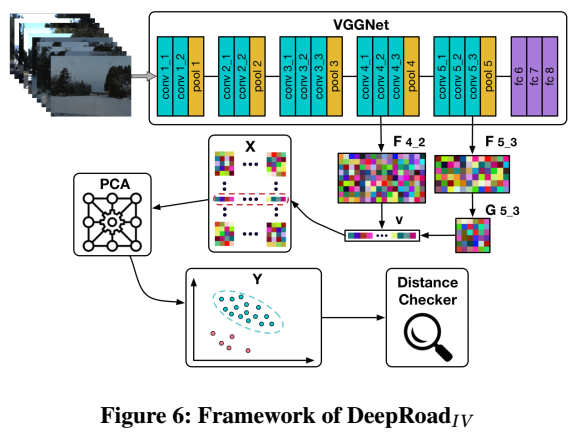# 实验

## 1. 度量

### 模型不一致性的度量

在该工作中，自动驾驶系统被定义为在修改驾驶图像的天气条件之后，如果其转向角预测落入特定误差范围内，则动作被认为是一致的。

### 验证输入的度量

首先，为了平衡输入数据和训练数据，我们从在线驾驶场景中收集M个图像作为输入数据，并从训练数据集中随机选择M个图像作为训练数据。其次，为了估计距离更稳定，我们平均每个图像的前N个最小距离来观察预测动作的相似性。

## 2. 结果

### $DeepRoad_{MT}$

奇数列是原图，偶数列是生成的图像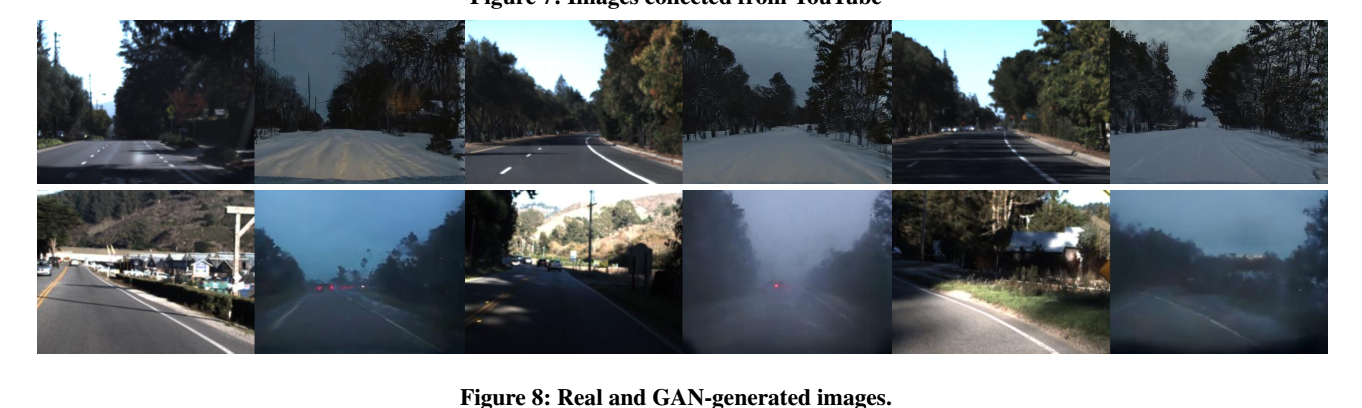当使用10°的误差界限和多雨的场景时，〖DeepRoad〗_MT分别为Autumn，Chauffeur和Rwightman三个模型检测到5279,710和656个不一致的行为。具体数据如下图：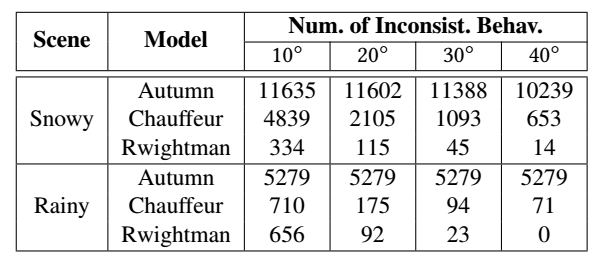具体误差的指出如下图：### $DeepRoad_{IV}$

图10显示了$DeepRoad_{IV}$在晴天，下雨和下雪的驾驶场景中的结果。具体而言，图10的前三个图分别呈现了晴天，下雨和下雪图像的结果。橙色和蓝色点表示采样训练和相应的输入图像。从图10a中，我们观察到大多数输入图像与训练样本混合，并且一些输入远离群集。从图10b和10c可以看出，输入点和训练点之间存在间隙，并且簇可以线性分离。这些结果表明，晴天和训练图像的分布是接近的，但是雨天和雪天的图像不是。另一方面，雨雪图像的群集相对紧凑，但是阳光图像是分散的。原因可能是雨雪图像的纹理是统一的，内容相对较差，因此图像之间的距离很小。然而，晴天图像的光照条件和内容更加多样化，因此距离很大。此外，从图10d可以看出，阳光图像的距离主要介于0和3之间，几乎所有雨雪图像的距离都大于2。假设输入验证的阈值为2.5，$DeepRoad_{IV}$可以检测到100％的雨天图像，85％的雪景图像和阳光图像中21％的异常值作为无效输入，有效地提高了系统的鲁棒性。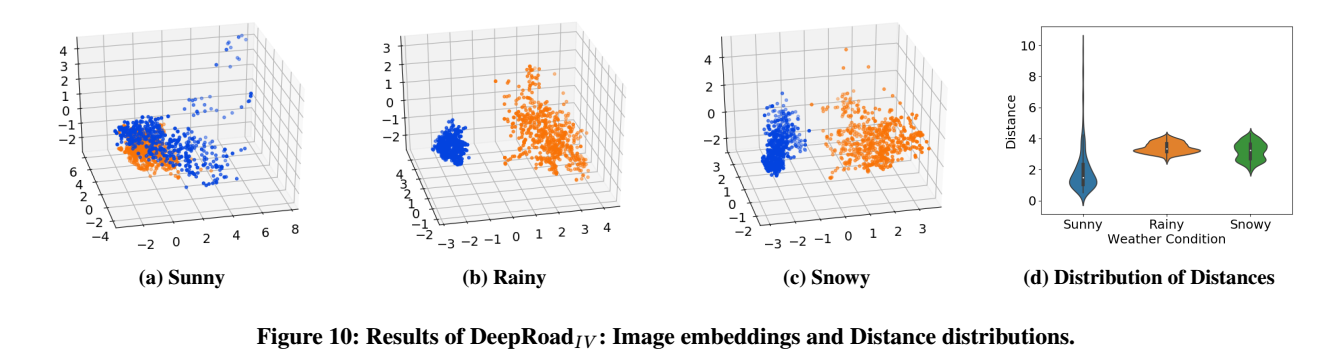此外，如下图所示，使用非线性转换是很有必要的。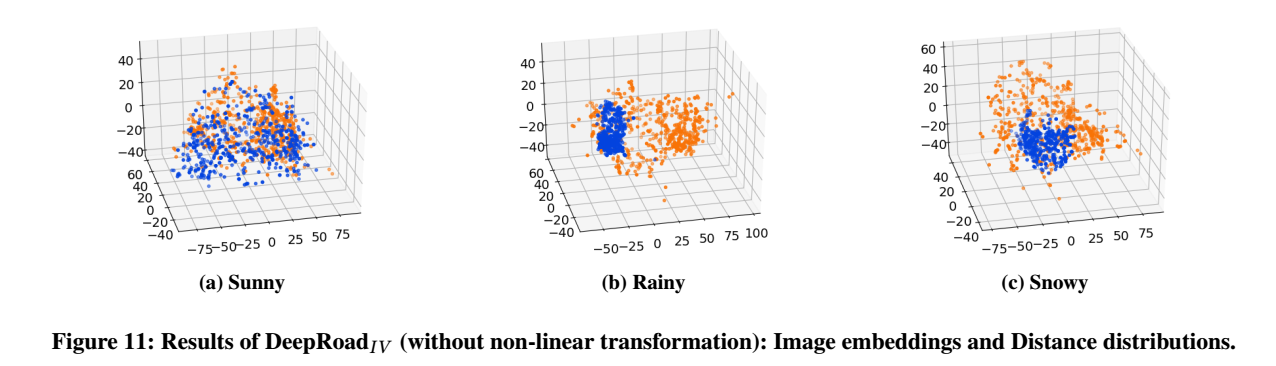##### Share